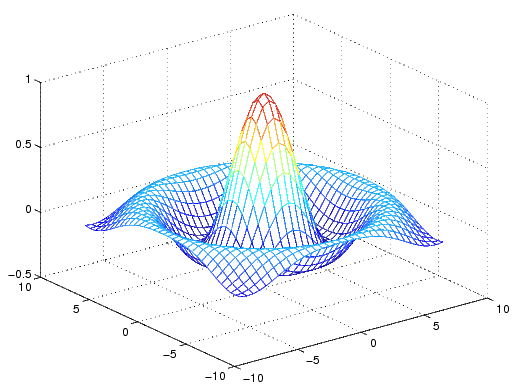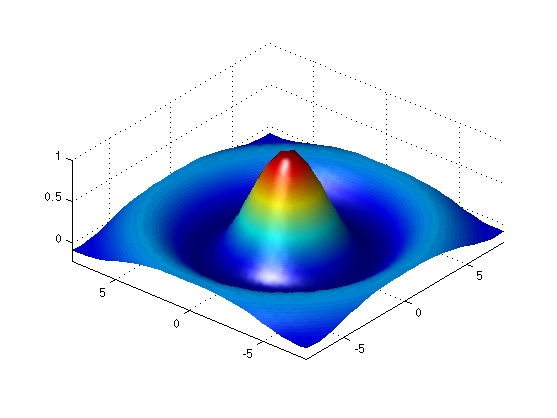3-D VisualizationVisualizing Functions of Two Variables

The first step in displaying a function of two variables, z = f(x,y), is to generate `X` and `Y` matrices consisting of repeated rows and columns, respectively, over the domain of the function. Then use these matrices to evaluate and graph the function.

The `meshgrid` function transforms the domain specified by two vectors, `x` and `y`, into matrices `X` and `Y`. You then use these matrices to evaluate functions of two variables. The rows of `X` are copies of the vector `x` and the columns of `Y` are copies of the vector `y`.

To illustrate the use of `meshgrid`, consider the `sin(r)/r` or `sinc` function. To evaluate this function between -8 and 8 in both x and y, you need pass only one vector argument to `meshgrid`, which is then used in both directions.

• ```[X,Y] = meshgrid(-8:.5:8);
R = sqrt(X.^2 + Y.^2) + eps;
```

The matrix `R` contains the distance from the center of the matrix, which is the origin. Adding `eps` prevents the divide by zero (in the next step) that produces `Inf` values in the data.

Forming the `sinc` function and plotting `Z` with `mesh` results in the 3-D surface.

• ```Z = sin(R)./R;
mesh(X,Y,Z)```

Emphasizing Surface Shape

MATLAB provides a number of techniques that can enhance the information content of your graphs. For example, this graph of the `sinc` function uses the same data as the previous graph, but employs lighting and view adjustment to emphasize the shape of the graphed function (`daspect`, `axis`, `view`, `camlight`).

• ```surf(X,Y,Z,'FaceColor','interp',...
'EdgeColor','none',...
'FaceLighting','phong')
daspect([5 5 1])
axis tight
view(-50,30)
camlight left```

See the `surf` function for more information on surface plots.Mesh and Surface Plots Surface Plots of Nonuniformly Sampled Data